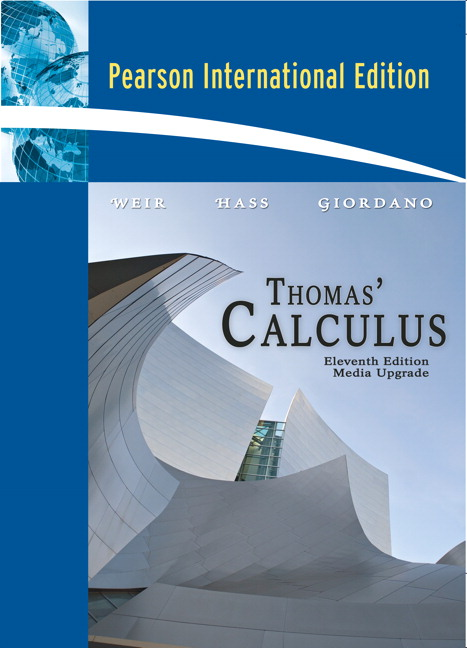# Joel Hass

## Books

#### Calculus Textbooks

I contributed to the classic calculus text Thomas' Calculus, starting with the 11th edition.
This textbook has several versions. In order of publication date they are:1. The standard 11th edition of Thomas' Calculus (2004).
2. The Early Transcendentals 11th edition of Thomas' Calculus (2005). (In this book the trigononometric, exponential and logarithm functions are treated sooner than in the standard version.)
3. The international 11th edition of Thomas' Calculus.1. The standard 1st edition of University Calculus (2006). University Calculus gives a more concise and focused treatment of calculus. This is an early transcendentals textbook.
2. The Alternate 1st edition of University Calculus (2007).
3. The 1st edition of University Calculus Elements (2008). Also early transcendentals. Even more concise.1. The 12th Edition of Thomas' Calculus (2009).
2. The Early Transcendentals version of Thomas' Calculus, 12th Edition (2009).
3. University Calculus, 2nd Edition (2011).1. The 13th Edition of Thomas' Calculus (2014).
2. The Early Transcendentals version of Thomas' Calculus, 13th Edition (2014).

#### Calculus study guides

I am a coauthor with Colin Adams and Abigail Thompson of the booksHow to Ace Calculus which appeared in 1998,
and the sequel
How to Ace the Rest of Calculus which appeared in 2001.